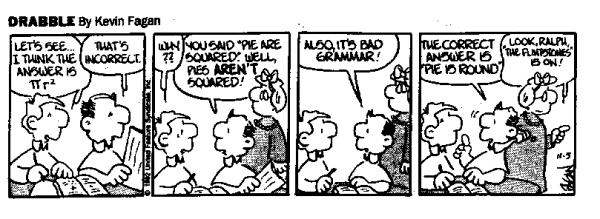### A very brief story of the PiWarped by Mike Cavna via Bamdad's Math Comics
As you know, the $\pi$ is defined as the ratio of the circumference to its diameter. This number, which is transcendental, was, apparently, known since ancient times. There are, in fact, some Egyptologists who believe that $\pi$, or perhaps $\tau = 2 \pi$, was known to them since the age og the Giza's pyramid, built between 2589 and 2566 BC, because the relationship between the perimeter and the height is 6.2857.
There are no explicit proof of the fact that, at the time, Egyptian mathematics became aware of a number such as $\pi$, however, between 600 and 1000 years later on a Babylonian tablet it is geometrically established the first value of $\pi$: $25/8 = 3.1250$. From documents written more or less in the same period it can be deduced that also the Egyptians calculated the value of $\pi$, obtaining $(16/9)^2 \simeq 3.1605$.
Indian mathematics, however, seems a little late: in 600 BC on Shulba Sutras, it is calculated for the $\pi$ value like $(9785/5568)^2 \simeq 3.088$, which will be updated later in 150 BC as $\sqrt{10} \simeq 3.1622$, which is a value much closer to the value calculated by the Egyptians.
A good approximation of $\pi$ value is in Mishnat ha-Middot, a geometric treatise by Rabbi Nehemiah: $3 + 1/7 \simeq 3.14286$.
The approximation, however, the most amazing not only for accuracy but also for the method is that proposed by Archimedes, the italo-greek mathematician who invented the method of polygons in order to calculate $\pi$, a costant that for a millennium became known simply as the Archimedes' constant.
He simply calculated the perimeter of polygons inscribed and circumscribed in a circle, thus obtaining a lower and an upper estimate of the value of the constant: $223/71 < \pi < 22/7$ or $3.1408 < \pi < 3.1429$ It's clear that his method of calculation is very modern and above suggests that Archimedes was well aware of the transcendental nature of the constant, which could be known only through approximations.
Today $\pi$ is known to 5 trillion digits and if you try to type the symbol $\pi$ on modern scientific calculators, the value they give you is, to the first decimal place, 3.14159265...3.1416? by Thaves via Bamdad's Math Comics
Calculating $\pi$ digits is like a mathematical art, that combines the technique of iterative algorithms with the convergent series. For example the achievment of 5 trilions of digits it could be possible thanks to Chudnovsky formula: $\frac{1}{\pi} = 12 \sum^\infty_{k=0} \frac{(-1)^k (6k)! (13591409 + 545140134k)}{(3k)!(k!)^3 640320^{3k + 3/2}}$ Alexander J. Yee and Shigeru Kondo, the two recordmen, they also used for controls the Plouffe formula or the BaileyBorweinPlouffe formula: $\pi = \sum_{k = 0}^{\infty} \frac{1}{16^k} \left( \frac{4}{8k + 1} - \frac{2}{8k + 4} - \frac{1}{8k + 5} - \frac{1}{8k + 6}\right)$ and the Bellard formula: $\pi=\frac{1}{2^6}\sum_{n=0}^\infty \frac{(-1)^n}{2^{10n}} \left (-\frac{2^5}{4n+1}-\frac{1}{4n+3}+\frac{2^8}{10n+1}\right.$ $\left.-\frac{2^6}{10n+3}-\frac{2^2}{10n+5}-\frac{2^2}{10n+7}+\frac{1}{10n+9}\right )$ It's interesting to observe that the previous series are based, in some way, to the series developed since 1914 by Srinivasa Ramanujan. One of the most famous and fast Ramanujan's series is: $\frac{1}{\pi} = \frac{2 \sqrt{2}}{9801} \sum_{k=0}^{\infty} \frac{(4k!) (1103 + 26390k)}{(k!)^4 396^{4k}}$ Other mathematicians developed other series, but one of the most curious is the Gregorys's series, which has the unfortunate flaw of being wrong only in a few of digits, for example the 6th, 11st, 12nd, 23rd, ... The other digits are correct! $\pi = 4 \sum_{k=1}^{500000} \frac{(-1)^{k-1}}{2k - 1}$ The last series that I would propose you is dued by Stanley Rabinowitz and Stan Wagon $\frac{\pi}{2} = \sum_{i=0}^\infty \frac{i!}{(2i+1)!!}$ where $k!!$ is the product of all the odd numbers up to $k$.It's a fractal! via Super Glitch
Aaron Klebanoff writes:
The Mandelbrot set is arguably one of the most beautiful sets in mathematics. In 1991, Dave Boll discovered a surprising occurrence of the number $\pi$ while exploring a seemingly unrelated property of the Mandelbrot set. Boll's finding is easy to describe and understand, and yet it is not widely known - possibly because the result has not been rigorously shown.
Boll is a student of computer science at Colorado State University and he is interested in fractals. And just playing with fractals, he comes across in his curious discovery. The young man, in fact, try to determine whether the Mandelbrot set is restricted in an infinite way, and so, given a small number $\varepsilon$, he calculated the product between $\sqrt{\varepsilon}$ and the number of iterations $N (\varepsilon)$ needed to zero orbit. And this product, for the limit of $\varepsilon$ smaller, coincides with $\pi$! $\lim_{\varepsilon \rightarrow 0^+} \sqrt{\varepsilon} N(\varepsilon) = \pi$ Klebanoff, at this point, tryes to rigorously proof Boll's discover, released through the student's web page:
Rather than attempt to complete the proof of Boll's vertical route, we do something much easier. We conjecture that there are infi nitely many such routes at each of the infi nitely many pinches of $M$.Drabble by Kevin Fagan via Bamdad's Math Comics

Chudnovsky D.V. (1989). The Computation of Classical Constants, Proceedings of the National Academy of Sciences, 86 (21) 8178-8182. DOI:
Bailey D., Borwein P. & Plouffe S. (1997). On the rapid computation of various polylogarithmic constants, Mathematics of Computation, 66 (218) 903-914. DOI:
Fabrice Bellard. Computation of the $n-th$ digit of pi in any base in $O(n^2)$
Borwein J.M. & Borwein P.B. (1988). Ramanujan and Pi, Scientific American, 258 (2) 112-117. DOI: (pdf)
Borwein J.M., Borwein P.B. & Dilcher K. (1989). Pi, Euler Numbers, and Asymptotic Expansions, The American Mathematical Monthly, 96 (8) 681. DOI: (pdf)
Rabinowitz S. & Wagon S. (1995). A Spigot Algorithm for the Digits of π, The American Mathematical Monthly, 102 (3) 195. DOI: (pdf)
Dave Boll, Pi and the Mandelbrot set
Klebanoff A. (2001). π in the Mandelbrot set, Fractals, 09 (04) 393-402. DOI:

Pi-links: Pi su en.wiki | Digits of Pi | Pi to 1,000,000 places